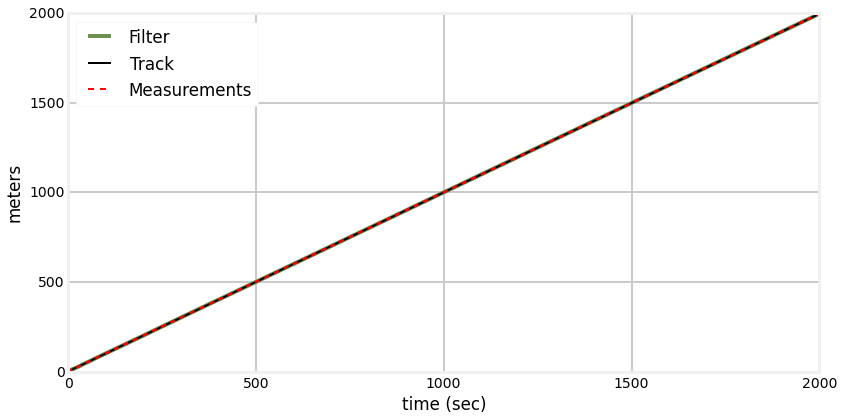# Designing Kalman Filters¶

In :
#format the book
%matplotlib inline
from __future__ import division, print_function
import matplotlib.pyplot as plt
import book_format

Out:

## Introduction¶

In this chapter we will work through the design of several Kalman filters to gain experience and confidence with the various equations and techniques.

For our first multidimensional problem we will track a robot in a 2D space, such as a field. We will start with a simple noisy sensor that outputs noisy $(x,y)$ coordinates which we will need to filter to generate a 2D track. Once we have mastered this concept, we will extend the problem significantly with more sensors and then adding control inputs. blah blah

## Tracking a Robot¶

This first attempt at tracking a robot will closely resemble the 1-D dog tracking problem of previous chapters. This will allow us to 'get our feet wet' with Kalman filtering. So, instead of a sensor that outputs position in a hallway, we now have a sensor that supplies a noisy measurement of position in a 2-D space, such as an open field. That is, at each time $T$ it will provide an $(x,y)$ coordinate pair specifying the measurement of the sensor's position in the field.

Implementation of code to interact with real sensors is beyond the scope of this book, so as before we will program simple simulations in Python to represent the sensors. We will develop several of these sensors as we go, each with more complications, so as I program them I will just append a number to the function name. pos_sensor1() is the first sensor we write, and so on.

So let's start with a very simple sensor, one that travels in a straight line. It takes as input the last position, velocity, and how much noise we want, and returns the new position.

In :
import numpy.random as random
import copy
class PosSensor1(object):
def __init__(self, pos = [0,0], vel = (0,0), noise_scale = 1.):
self.vel = vel
self.noise_scale = noise_scale
self.pos = copy.deepcopy(pos)

self.pos += self.vel
self.pos += self.vel

return [self.pos + random.randn() * self.noise_scale,
self.pos + random.randn() * self.noise_scale]


A quick test to verify that it works as we expect.

In :
import book_plots as bp

pos = [4,3]
sensor = PosSensor1 (pos, (2,1), 1)

for i in range (50):
bp.plot_measurements(pos, pos)

plt.show()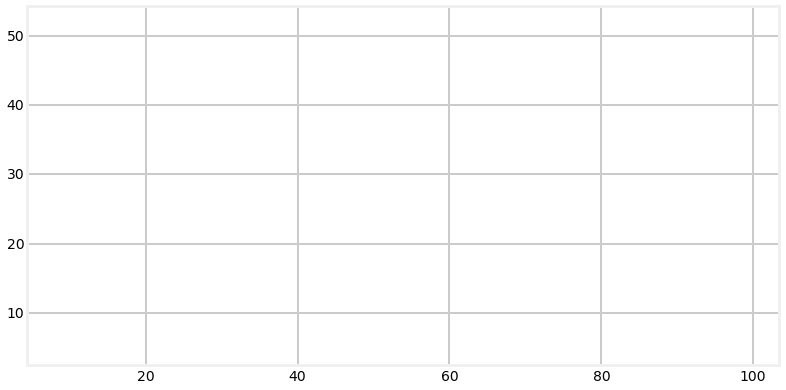That looks correct. The slope is 1/2, as we would expect with a velocity of (2,1), and the data seems to start at near (6,4).

##### Step 1: Choose the State Variables¶

As always, the first step is to choose our state variables. We are tracking in two dimensions and have a sensor that gives us a reading in each of those two dimensions, so we know that we have the two observed variables $x$ and $y$. If we created our Kalman filter using only those two variables the performance would not be very good because we would be ignoring the information velocity can provide to us. We will want to incorporate velocity into our equations as well. I will represent this as

$$\mathbf{x} = \begin{bmatrix}x\\v_x\\y\\v_y\end{bmatrix}$$

There is nothing special about this organization. I could have listed the (xy) coordinates first followed by the velocities, and/or I could done this as a row matrix instead of a column matrix. For example, I could have chosen:

$$\mathbf{x} = \begin{bmatrix}x&y&v_x&v_y\end{bmatrix}$$

All that matters is that the rest of my derivation uses this same scheme. However, it is typical to use column matrices for state variables, and I prefer it, so that is what we will use.

It might be a good time to pause and address how you identify the unobserved variables. This particular example is somewhat obvious because we already worked through the 1D case in the previous chapters. Would it be so obvious if we were filtering market data, population data from a biology experiment, and so on? Probably not. There is no easy answer to this question. The first thing to ask yourself is what is the interpretation of the first and second derivatives of the data from the sensors. We do that because obtaining the first and second derivatives is mathematically trivial if you are reading from the sensors using a fixed time step. The first derivative is just the difference between two successive readings. In our tracking case the first derivative has an obvious physical interpretation: the difference between two successive positions is velocity.

Beyond this you can start looking at how you might combine the data from two or more different sensors to produce more information. This opens up the field of sensor fusion, and we will be covering examples of this in later sections. For now, recognize that choosing the appropriate state variables is paramount to getting the best possible performance from your filter.

##### Step 2: Design State Transition Function¶

Our next step is to design the state transition function. Recall that the state transition function is implemented as a matrix $\mathbf{F}$ that we multipy with the previous state of our system to get the next state, like so.

$$\mathbf{x}' = \mathbf{Fx}$$

I will not belabor this as it is very similar to the 1-D case we did in the previous chapter. Our state equations for position and velocity would be:

\begin{aligned} x' &= (1*x) + (\Delta t * v_x) + (0*y) + (0 * v_y) \\ v_x &= (0*x) + (1*v_x) + (0*y) + (0 * v_y) \\ y' &= (0*x) + (0* v_x) + (1*y) + (\Delta t * v_y) \\ v_y &= (0*x) + (0*v_x) + (0*y) + (1 * v_y) \end{aligned}

Laying it out that way shows us both the values and row-column organization required for $\small\mathbf{F}$. In linear algebra, we would write this as:

$$\begin{bmatrix}x\\v_x\\y\\v_y\end{bmatrix}' = \begin{bmatrix}1& \Delta t& 0& 0\\0& 1& 0& 0\\0& 0& 1& \Delta t\\ 0& 0& 0& 1\end{bmatrix}\begin{bmatrix}x\\v_x\\y\\v_y\end{bmatrix}$$

So, let's do this in Python. It is very simple; the only thing new here is setting dim_z to 2. We will see why it is set to 2 in step 4.

In :
from filterpy.kalman import KalmanFilter
import numpy as np

tracker = KalmanFilter(dim_x=4, dim_z=2)
dt = 1.   # time step

tracker.F = np.array ([[1, dt, 0,  0],
[0,  1, 0,  0],
[0,  0, 1, dt],
[0,  0, 0,  1]])

##### Step 3: Design the Motion Function¶

We have no control inputs to our robot (yet!), so this step is trivial - set the motion input $\small\mathbf{u}$ to zero. This is done for us by the class when it is created so we can skip this step, but for completeness we will be explicit.

In :
tracker.u = 0.

##### Step 4: Design the Measurement Function¶

The measurement function defines how we go from the state variables to the measurements using the equation $\mathbf{z} = \mathbf{Hx}$. At first this is a bit counterintuitive, after all, we use the Kalman filter to go from measurements to state. But the update step needs to compute the residual between the current measurement and the measurement represented by the prediction step. Therefore $\textbf{H}$ is multiplied by the state $\textbf{x}$ to produce a measurement $\textbf{z}$.

In this case we have measurements for (x,y), so $\textbf{z}$ must be of dimension $2\times 1$. Our state variable is size $4\times 1$. We can deduce the required size for $\textbf{H}$ by recalling that multiplying a matrix of size $m\times n$ by $n\times p$ yields a matrix of size $m\times p$. Thus,

\begin{aligned} (2\times 1) &= (a\times b)(4 \times 1) \\ &= (a\times 4)(4\times 1) \\ &= (2\times 4)(4\times 1) \end{aligned}

So, $\textbf{H}$ is of size $2\times 4$.

Filling in the values for $\textbf{H}$ is easy in this case because the measurement is the position of the robot, which is the $x$ and $y$ variables of the state $\textbf{x}$. Let's make this just slightly more interesting by deciding we want to change units. So we will assume that the measurements are returned in feet, and that we desire to work in meters. Converting from feet to meters is a simple as multiplying by 0.3048. However, we are converting from state (meters) to measurements (feet) so we need to divide by 0.3048. So

$$\mathbf{H} = \begin{bmatrix} \frac{1}{0.3048} & 0 & 0 & 0 \\ 0 & 0 & \frac{1}{0.3048} & 0 \end{bmatrix}$$

which corresponds to these linear equations \begin{aligned} z_x' &= (\frac{x}{0.3048}) + (0* v_x) + (0*y) + (0 * v_y) \\ z_y' &= (0*x) + (0* v_x) + (\frac{y}{0.3048}) + (0 * v_y) \\ \end{aligned}

To be clear about my intentions here, this is a pretty simple problem, and we could have easily found the equations directly without going through the dimensional analysis that I did above. In fact, an earlier draft did just that. But it is useful to remember that the equations of the Kalman filter imply a specific dimensionality for all of the matrices, and when I start to get lost as to how to design something it is often extremely useful to look at the matrix dimensions. Not sure how to design $\textbf{H}$? Here is the Python that implements this:

In :
tracker.H = np.array([[1/0.3048, 0, 0, 0],
[0, 0, 1/0.3048, 0]])
print(tracker.H)

[[ 3.2808399  0.         0.         0.       ]
[ 0.         0.         3.2808399  0.       ]]

##### Step 5: Design the Measurement Noise Matrix¶

In this step we need to mathematically model the noise in our sensor. For now we will make the simple assumption that the $x$ and $y$ variables are independent Gaussian processes. That is, the noise in x is not in any way dependent on the noise in y, and the noise is normally distributed about the mean. For now let's set the variance for $x$ and $y$ to be 5 for each. They are independent, so there is no covariance, and our off diagonals will be 0. This gives us:

$$\mathbf{R} = \begin{bmatrix}5&0\\0&5\end{bmatrix}$$

It is a $2{\times}2$ matrix because we have 2 sensor inputs, and covariance matrices are always of size $n{\times}n$ for $n$ variables. In Python we write:

In :
tracker.R = np.array([[5, 0],
[0, 5]])
print(tracker.R)

[[5 0]
[0 5]]

##### Step 6: Design the Process Noise Matrix¶

Finally, we design the process noise. We don't yet have a good way to model process noise, so for now we will assume there is a small amount of process noise, say 0.1 for each state variable. Later we will tackle this admittedly difficult topic in more detail. We have 4 state variables, so we need a $4{\times}4$ covariance matrix:

$$\mathbf{Q} = \begin{bmatrix}0.1&0&0&0\\0&0.1&0&0\\0&0&0.1&0\\0&0&0&0.1\end{bmatrix}$$

In Python I will use the numpy eye helper function to create an identity matrix for us, and multipy it by 0.1 to get the desired result.

In :
tracker.Q = np.eye(4) * 0.1
print(tracker.Q)

[[ 0.1  0.   0.   0. ]
[ 0.   0.1  0.   0. ]
[ 0.   0.   0.1  0. ]
[ 0.   0.   0.   0.1]]


For our simple problem we will set the initial position at (0,0) with a velocity of (0,0). Since that is a pure guess, we will set the covariance matrix $\small\mathbf{P}$ to a large value. $$\mathbf{x} = \begin{bmatrix}0\\0\\0\\0\end{bmatrix}\\ \mathbf{P} = \begin{bmatrix}500&0&0&0\\0&500&0&0\\0&0&500&0\\0&0&0&500\end{bmatrix}$$

In Python we implement that with

In :
tracker.x = np.array([[0, 0, 0, 0]]).T
tracker.P = np.eye(4) * 500.
print(tracker.x)
print()
print (tracker.P)

[[ 0.]
[ 0.]
[ 0.]
[ 0.]]

[[ 500.    0.    0.    0.]
[   0.  500.    0.    0.]
[   0.    0.  500.    0.]
[   0.    0.    0.  500.]]


## Implement the Filter Code¶

Design is complete, now we just have to write the Python code to run our filter, and output the data in the format of our choice. To keep the code clear, let's just print a plot of the track. We will run the code for 30 iterations.

In :
tracker = KalmanFilter(dim_x=4, dim_z=2)
dt = 1.0   # time step

tracker.F = np.array ([[1, dt, 0,  0],
[0,  1, 0,  0],
[0,  0, 1, dt],
[0,  0, 0,  1]])
tracker.u = 0.
tracker.H = np.array ([[1/0.3048, 0, 0, 0],
[0, 0, 1/0.3048, 0]])

tracker.R = np.eye(2) * 5
tracker.Q = np.eye(4) * .1

tracker.x = np.array([[0,0,0,0]]).T
tracker.P = np.eye(4) * 500.

# initialize storage and other variables for the run
count = 30
xs, ys = [],[]
pxs, pys = [],[]

sensor = PosSensor1 ([0,0], (2,1), 1.)

for i in range(count):
z = np.array([[pos], [pos]])

tracker.predict ()
tracker.update (z)

xs.append (tracker.x[0,0])
ys.append (tracker.x[2,0])
pxs.append (pos*.3048)
pys.append(pos*.3048)

bp.plot_filter(xs, ys)
bp.plot_measurements(pxs, pys)
plt.legend(loc=2)
plt.xlim((0,20))
plt.show()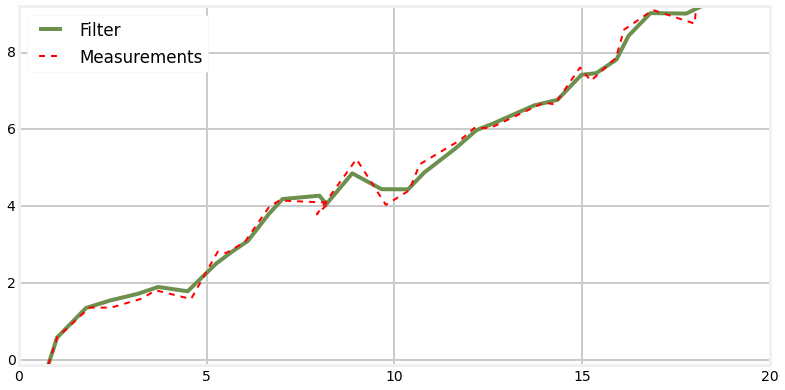I encourage you to play with this, setting $\mathbf{Q}$ and $\mathbf{R}$ to various values. However, we did a fair amount of that sort of thing in the last chapters, and we have a lot of material to cover, so I will move on to more complicated cases where we will also have a chance to experience changing these values.

Now I will run the same Kalman filter with the same settings, but also plot the covariance ellipse for $x$ and $y$. First, the code without explanation, so we can see the output. I print the last covariance to see what it looks like. But before you scroll down to look at the results, what do you think it will look like? You have enough information to figure this out but this is still new to you, so don't be discouraged if you get it wrong.

In :
import stats

tracker = KalmanFilter(dim_x=4, dim_z=2)
dt = 1.0   # time step

tracker.F = np.array([[1, dt, 0,  0],
[0,  1, 0,  0],
[0,  0, 1, dt],
[0,  0, 0,  1]])
tracker.u = 0.
tracker.H = np.array([[1/0.3048, 0, 0, 0],
[0, 0, 1/0.3048, 0]])

tracker.R = np.eye(2) * 5
tracker.Q = np.eye(4) * .1

tracker.x = np.array([[0, 0, 0, 0]]).T
tracker.P = np.eye(4) * 500.

# initialize storage and other variables for the run
count = 30
xs, ys = [], []
pxs, pys = [], []

sensor = PosSensor1([0,0], (2,1), 1.)

for i in range(count):
z = np.array([[pos], [pos]])

tracker.predict()
tracker.update(z)

xs.append(tracker.x[0,0])
ys.append(tracker.x[2,0])
pxs.append(pos*.3048)
pys.append(pos*.3048)

# plot covariance of x and y
cov = np.array([[tracker.P[0,0], tracker.P[2,0]],
[tracker.P[0,2], tracker.P[2,2]]])

stats.plot_covariance_ellipse(
(tracker.x[0,0], tracker.x[2,0]), cov=cov,
facecolor='g', alpha=0.15)

bp.plot_filter(xs, ys)
bp.plot_measurements(pxs, pys)
plt.legend(loc=2)
plt.show()
print("final P is:")
print(tracker.P)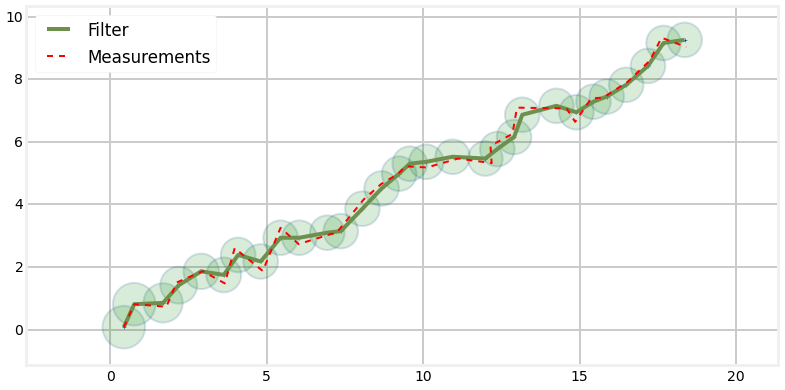final P is:
[[ 0.30660483  0.12566239  0.          0.        ]
[ 0.12566239  0.24399092  0.          0.        ]
[ 0.          0.          0.30660483  0.12566239]
[ 0.          0.          0.12566239  0.24399092]]


Did you correctly predict what the covariance matrix and plots would look like? Perhaps you were expecting a tilted ellipse, as in the last chapters. If so, recall that in those chapters we were not plotting $x$ against $y$, but $x$ against $\dot{x}$. $x$ is correlated to $\dot{x}$, but $x$ is not correlated or dependent on $y$. Therefore our ellipses are not tilted. Furthermore, the noise for both $x$ and $y$ are modeled to have the same value, 5, in $\mathbf{R}$. If we were to set R to, for example,

$$\mathbf{R} = \begin{bmatrix}10&0\\0&5\end{bmatrix}$$

we would be telling the Kalman filter that there is more noise in $x$ than $y$, and our ellipses would be longer than they are tall.

The final P tells us everything we need to know about the correlation between the state variables. If we look at the diagonal alone we see the variance for each variable. In other words $\mathbf{P}_{0,0}$ is the variance for x, $\mathbf{P}_{1,1}$ is the variance for $\dot{x}$, $\mathbf{P}_{2,2}$ is the variance for y, and $\mathbf{P}_{3,3}$ is the variance for $\dot{y}$. We can extract the diagonal of a matrix using numpy.diag().

In :
print(np.diag(tracker.P))

[ 0.30660483  0.24399092  0.30660483  0.24399092]


The covariance matrix contains four $2{\times}2$ matrices that you should be able to easily pick out. This is due to the correlation of $x$ to $\dot{x}$, and of $y$ to $\dot{y}$. The upper left hand side shows the covariance of $x$ to $\dot{x}$. Let's extract and print, and plot it.

In :
c = tracker.P[0:2, 0:2]
print(c)
stats.plot_covariance_ellipse((0, 0), cov=c, facecolor='g', alpha=0.2)

[[ 0.30660483  0.12566239]
[ 0.12566239  0.24399092]]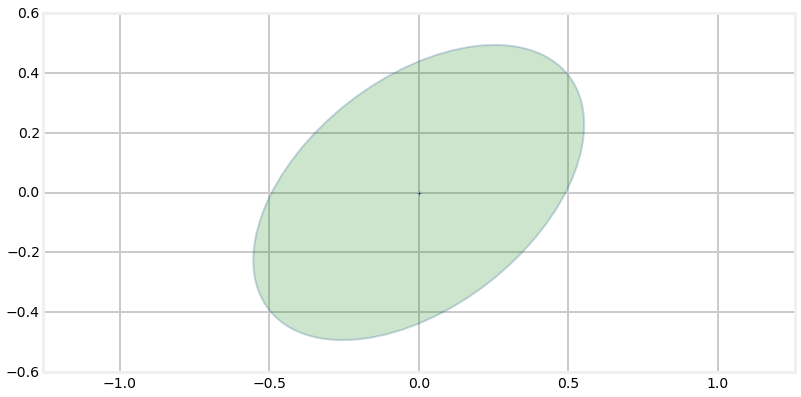The covariance contains the data for $x$ and $\dot{x}$ in the upper left because of how it is organized. Recall that entries $\mathbf{P}_{i,j}$ and $\mathbf{P}_{j,i}$ contain $p\sigma_1\sigma_2$.

Finally, let's look at the lower left side of $\mathbf{P}$, which is all 0s. Why 0s? Consider $\mathbf{P}_{3,0}$. That stores the term $p\sigma_3\sigma_0$, which is the covariance between $\dot{y}$ and $x$. These are independent, so the term will be 0. The rest of the terms are for similarly independent variables.

## The Effect of Order¶

So far in this book we have only really considered tracking position and velocity. That has worked well, but only because I have been carefully selecting problems for which this was an appropriate choice. You know have enough experience with the Kalman filter to consider this in more general terms.

What do I mean by "order"? In the context of these system models it is the number of derivatives required to accurately model a system. Consider a system that does not change, such as the height of a building. There is no change, so there is no need for a derivative, and the order of the system is zero. We could express this in an equation as

$$x = 312.5$$

A first order system has a first derivative. For example, change of position is velocity, and we can write this as

$$v = \frac{dx}{dt}$$

which we integrate into the Newton equation $$x = vt + x_0.$$

This is also called a constant velocity model, because of the assumption of a constant velocity.

So a second order has a second derivative. The second derivative of position is acceleration, with the equation

$$a = \frac{d^2x}{dt^2}$$

which we integrate into

$$x = \frac{1}{2}at^2 +v_0t + x_0.$$

This is also known as a constant acceleration model.

Another, equivalent way of looking at this is to consider the order of the polynomial. The constant acceleration model has a second derivative, so it is second order. Likewise, the polynomial $x = \frac{1}{2}at^2 +v_0t + x_0$ is second order.

When we design the state variables and process model we must choose the order of the system we want to model. Let's say we are tracking something with a constant velocity. No real world process is perfect, and so there will be slight variations in the velocity over short time period. You might reason that the best approach is to use a second order filter, allowing the acceleration term to deal with the slight variations in velocity.

That doesn't work as nicely as you might think. To thoroughly undestand this issue lets see the effects of using a process model that does not match the order of the system being filtered.

First we need a system to filter. I'll write a class to simulate on object with a constant velocity. Essentially no physical system has a truly constant velocity, so on each update we alter the velocity by a small amount. I also write a sensor to simulate Gaussian noise in a sensor. The code is below, and a plot an example run to verify that it is working correctly.

In :
from numpy.random import randn
import numpy as np
import matplotlib.pyplot as plt
from book_plots import plot_track

np.random.seed(124)
class ConstantVelocityObject(object):
def __init__(self, x0=0, vel=1., noise_scale=0.06):
self.x = x0
self.vel = vel
self.noise_scale = noise_scale

def update(self):
self.vel += randn()*self.noise_scale
self.x += self.vel
return (self.x, self.vel)

def sense(x, noise_scale=1.):
return x + randn()*noise_scale

obj = ConstantVelocityObject()

xs = []
zs = []
for i in range(50):
x = obj.update()
z = sense(x)
xs.append(x)
zs.append(z)

xs = np.asarray(xs)
bp.plot_track(xs[:,0])
bp.plot_measurements(range(50), zs)
plt.legend(loc='best')
plt.show()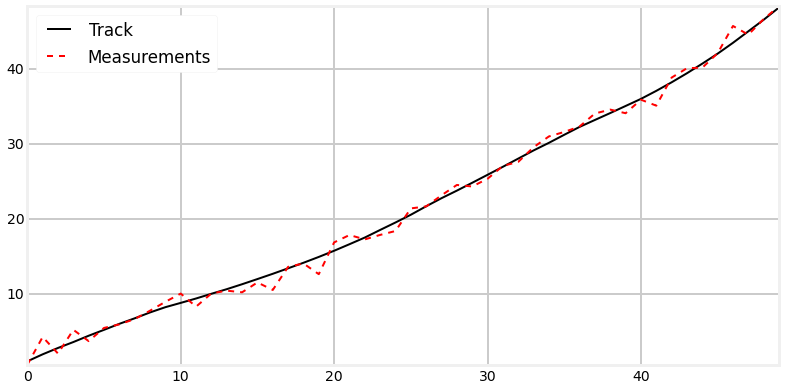I am satified with this plot. The track is not perfectly straight due to the noise that we added to the system - this could be the track of a person walking down the street, or perhaps of an aircraft being buffeted by variable winds. There is no intentional acceleration here, so we call it a constant velocity system. Again, you may be asking yourself that since there is in fact a tiny bit of acceleration going on why would we not use a second order Kalman filter to account for those changes? Let's find out.

How does one design a zero order, first order, or second order Kalman filter. We have been doing it all along, but just not using those terms. It might be slightly tedious, but I will elaborate fully on each - if the concept is clear to you feel free to skim a bit. However, I think that reading carefully will really cement the idea of filter order in your mind.

### Zero Order Kalman Filter¶

A zero order Kalman filter is just a filter that tracks with no derivatives. We are tracking position, so that means we only have a state variable for position (no velocity or acceleration), and the state transition function also only accounts for position. Using the matrix formulation we would say that the state variable is

$$\mathbf{x} = \begin{bmatrix}x\end{bmatrix}$$

The state transition function is very simple. There is no change in position, so we need to model $x=x$; in other words, x at time t+1 is the same as it was at time t. In matrix form, our state transition function is

$$\mathbf{F} = \begin{bmatrix}1\end{bmatrix}$$

The measurement function is very easy. Recall that we need to define how to convert the state variable $\mathbf{x}$ into a measurement. We will assume that our measurements are positions. The state variable only contains a position, so we get

$$\mathbf{H} = \begin{bmatrix}1\end{bmatrix}$$

That is pretty much it. Let's write a function that constructs and returns a zero order Kalman filter to us.

In :
def ZeroOrderKF(R, Q):
""" Create zero order Kalman filter. Specify R and Q as floats."""
kf = KalmanFilter(dim_x=1, dim_z=1)
kf.x = np.array([0.])
kf.R *= R
kf.Q *= Q
kf.P *= 20
kf.F = np.array([[1.]])
kf.H = np.array([[1.]])
return kf


### First Order Kalman Filter¶

A first order Kalman filter tracks a first order system, such as position and velocity. We already did this for the dog tracking problem above, so this should be very clear. But let's do it again.

A first order system has position and velocity, so the state variable needs both of these. The matrix formulation could be

$$\mathbf{x} = \begin{bmatrix}x\\\dot{x}\end{bmatrix}$$

As an aside, there is nothing stopping us from choosing

$$\mathbf{x} = \begin{bmatrix}\dot{x}\\x\end{bmatrix}$$

but all texts and software that I know of choose the first form as more natural. You would just have to design the rest of the matrices to take this ordering into account.

So now we have to design our state transition. The Newtonian equations for a time step are:

\begin{aligned} x_t &= x_{t-1} + v\Delta t \\ v_t &= v_{t-1}\end{aligned}

Recall that we need to convert this into the linear equation

$$\begin{bmatrix}x\\\dot{x}\end{bmatrix} = \mathbf{F}\begin{bmatrix}x\\\dot{x}\end{bmatrix}$$

Setting

$$\mathbf{F} = \begin{bmatrix}1 &\Delta t\\ 0 & 1\end{bmatrix}$$

gives us the equations above. If this is not clear, work out the matrix multiplication:

$$x = 1x + dt \dot{x} \\ \dot{x} = 0x + 1\dot{x}$$

Finally, we design the measurement function. The measurement function needs to implement

$$z = \mathbf{Hx}$$

Our sensor still only reads position, so it should take the position from the state, and 0 out the velocity, like so:

$$\mathbf{H} = \begin{bmatrix}1 & 0 \end{bmatrix}$$

As in the previous section we will define a function that constructs and returns a Kalman filter that implements these equations.

In :
from filterpy.common import Q_discrete_white_noise

def FirstOrderKF(R, Q, dt):
""" Create zero order Kalman filter. Specify R and Q as floats."""
kf = KalmanFilter(dim_x=2, dim_z=1)
kf.x = np.zeros(2)
kf.P *= np.array([[100,0], [0,1]])
kf.R *= R
kf.Q = Q_discrete_white_noise(2, dt, Q)
kf.F = np.array([[1., dt],
[0. , 1]])
kf.H = np.array([[1., 0]])
return kf


### Second Order Kalman Filter¶

A second order Kalman filter tracks a second order system, such as position, velocity and acceleration. The state variables will need to contain all three. The matrix formulation could be

$$\mathbf{x} = \begin{bmatrix}x\\\dot{x}\\\ddot{x}\end{bmatrix}$$

So now we have to design our state transition. The Newtonian equations for a time step are:

\begin{aligned} x_t &= x_{t-1} + v\Delta t + 0.5a_{t-1} \Delta t^2 \\ v_t &= v_{t-1} \Delta t + a_{t-1} \\ a_t &= a_{t-1}\end{aligned}

Recall that we need to convert this into the linear equation

$$\begin{bmatrix}x\\\dot{x}\\\ddot{x}\end{bmatrix} = \mathbf{F}\begin{bmatrix}x\\\dot{x}\\\ddot{x}\end{bmatrix}$$

Setting

$$\mathbf{F} = \begin{bmatrix}1 & \Delta t &.5\Delta t^2\\ 0 & 1 & \Delta t \\ 0 & 0 & 1\end{bmatrix}$$

gives us the equations above.

Finally, we design the measurement function. The measurement function needs to implement

$$z = \mathbf{Hx}$$

Our sensor still only reads position, so it should take the position from the state, and 0 out the velocity, like so:

$$\mathbf{H} = \begin{bmatrix}1 & 0 & 0\end{bmatrix}$$

As in the previous section we will define a function that constructs and returns a Kalman filter that implements these equations.

In :
def SecondOrderKF(R_std, Q, dt):
""" Create zero order Kalman filter. Specify R and Q as floats."""
kf = KalmanFilter(dim_x=3, dim_z=1)
kf.x = np.zeros(3)
kf.P[0,0] = 100
kf.P[1,1] = 1
kf.P[2,2] = 1
kf.R *= R_std**2
kf.Q = Q_discrete_white_noise(3, dt, Q)
kf.F = np.array([[1., dt, .5*dt*dt],
[0., 1.,       dt],
[0., 0.,       1.]])
kf.H = np.array([[1., 0., 0.]])
return kf


### Evaluating the Performance¶

We have implemented the Kalman filters and the simulated first order system, so now we can run each Kalman filter against the simulation and evaluate the results.

How do we evaluate the results? We can do this qualitatively by plotting the track and the Kalman filter output and eyeballing the results. However, we can do this far more rigorously with mathematics. Recall that system covariance matrix $\mathbf{P}$ contains the computed variance and covariances for each of the state variables. The diagonal contains the variance. If you think back to the Gaussian chapter you'll remember that roughly 99% of all measurements fall within three standard deviations if the noise is Gaussian, and, of course, the standard deviation can be computed as the square root of the variance. If this is not clear please review the Gaussian chapter before continuing, as this is an important point.

So we can evaluate the filter by looking at the residuals between the estimated state and actual state and comparing them to the standard deviations which we derive from $\mathbf{P}$. If the filter is performing correctly 99% of the residuals will fall within the third standard deviation. This is true for all the state variables, not just for the position.

So let's run the first order Kalman filter against our first order system and access it's performance. You can probably guess that it will do well, but let's look at it using the stardard deviations.

First, let's write a routine to generate the noisy measurements for us.

In :
def simulate_system(Q, count):
obj = ConstantVelocityObject(x0=0, vel=1, noise_scale=Q)
zs = []
xs = []
for i in range(count):
x = obj.update()
z = sense(x)
xs.append(x)
zs.append(z)
return np.asarray(xs), zs


And now a routine to perform the filtering.

In :
def filter_data(kf, zs):
# save output for plotting
fxs = []
ps = []

for z in zs:
kf.predict()
kf.update(z)

fxs.append(kf.x)
ps.append(kf.P.diagonal())

fxs = np.asarray(fxs)
ps = np.asarray(ps)

return fxs, ps


And to plot the track results.

In :
def plot_kf_output(xs, filter_xs, zs, title=None):
bp.plot_filter(filter_xs[:,0])
bp.plot_track(xs[:,0])

if zs is not None:
bp.plot_measurements(zs)
plt.legend(loc='best')
plt.ylabel('meters')
plt.xlabel('time (sec)')
if title is not None:
plt.title(title)
plt.xlim((-1, len(xs)))
plt.ylim((-1, len(xs)))
#plt.axis('equal')
plt.show()


Now we are prepared to run the filter and look at the results.

In :
R = 1
Q = 0.03

xs, zs = simulate_system(Q=Q, count=50)

kf = FirstOrderKF(R, Q, dt=1)
fxs1, ps1 = filter_data(kf, zs)

plt.figure()
plot_kf_output(xs, fxs1, zs)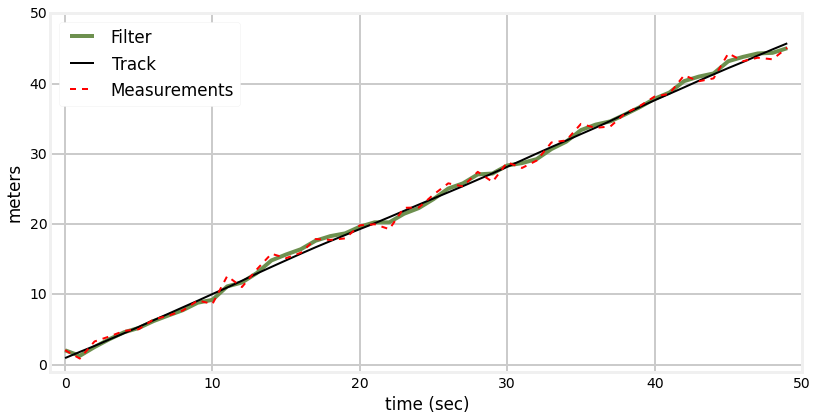It looks like the filter is performing well, but it is hard to tell exactly how well. Let's look at the residuals and see if they help. You may have noticed that in the code above I saved the covariance at each step. I did that to use in the following plot. The ConstantVelocityObject class returns a tuple of (position, velocity) for the real object, and this is stored in the array xs, and the filter's estimates are in fxs.

In :
def plot_residuals(xs, filter_xs, Ps, title, y_label):
res = xs - filter_xs
plt.plot(res)
bp.plot_residual_limits(Ps)
plt.title(title)
plt.ylabel(y_label)
plt.xlabel('time (sec)')

plt.show()

In :
plot_residuals(xs[:,0], fxs1[:,0], ps1[:,0],
'First Order Position Residuals',
'meters')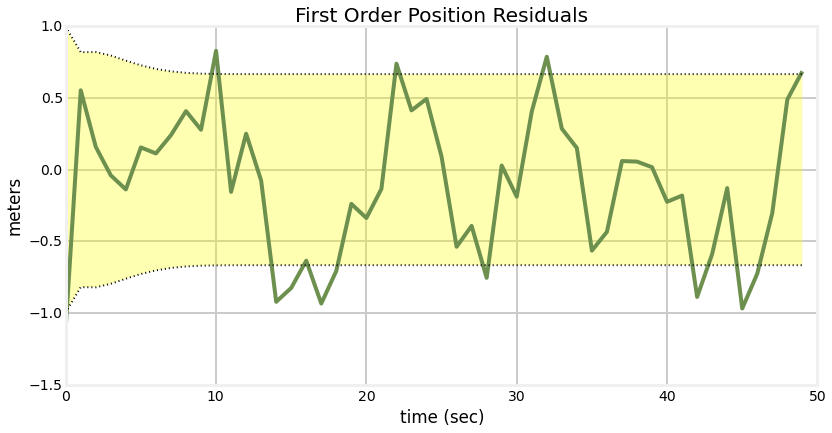How do we interpret this plot? The residual is drawn as the jagged line - the difference between the measurement and the actual position. If there was no measurement noise and the Kalman filter prediction was always perfect the residual would always be zero. So the ideal output would be a horizontal line at 0. We can see that the residual is centered around 0, so this gives us confidence that the noise is Gaussian (because the errors fall equally above and below 0). The yellow area between dotted lines show the theoretical performance of the filter for 1 standard deviations. In other words, approximately 68% of the errors should fall within the dotted lines. The residual falls within this range, so we see that the filter is performing well, and that it is not diverging.

But that is just for position. Let's look at the residuals for velocity.

In :
plot_residuals(xs[:,1], fxs1[:,1], ps1[:,1],
'First Order Velocity Residuals',
'meters/sec')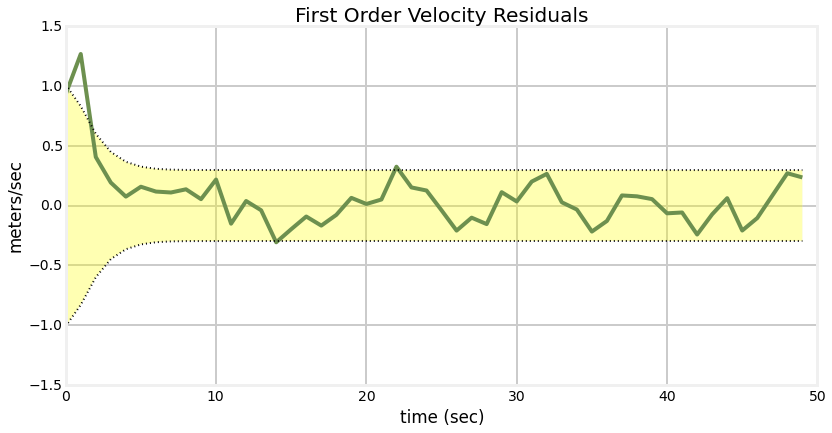Again, as expected, the residual falls within the theoretical performance of the filter, so we feel confident that the filter is well designed for this system.

Now let's do the same thing using the zero order Kalman filter. All of the code and math is largely the same, so let's just look at the results without discussing the implementation much.

In :
kf0 = ZeroOrderKF(R, Q)
fxs0, ps0 = filter_data(kf0, zs)

plot_kf_output(xs, fxs0, zs)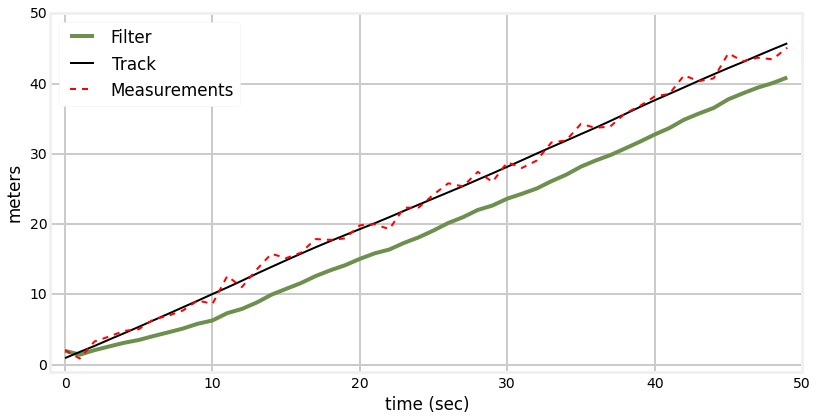As we would expect, the filter has problems. Think back to the g-h filter, where we incorporated acceleration into the system. The g-h filter always lagged the input because there were not enough terms to allow the filter to adjust quickly enough to the changes in velocity. The same thing is happening here, just one order lower. On every predict() step the Kalman filter assumes that there is no change in position - if the current position is 4.3 it will predict that the position at the next time period is 4.3. Of course, the actual position is closer to 5.3. The measurment, with noise, might be 5.4, so the filter chooses an estimate part way between 4.3 and 5.4, causing it to lag the actual value of 5.3 by a significant amount. This same thing happens in the next step, the next one, and so on. The filter never catches up.

Now let's look at the residuals. We are not tracking velocity, so we can only look at the residual for position.

In :
plot_residuals(xs[:,0], fxs0[:,0], ps0[:,0],
'Zero Order Position Residuals',
'meters')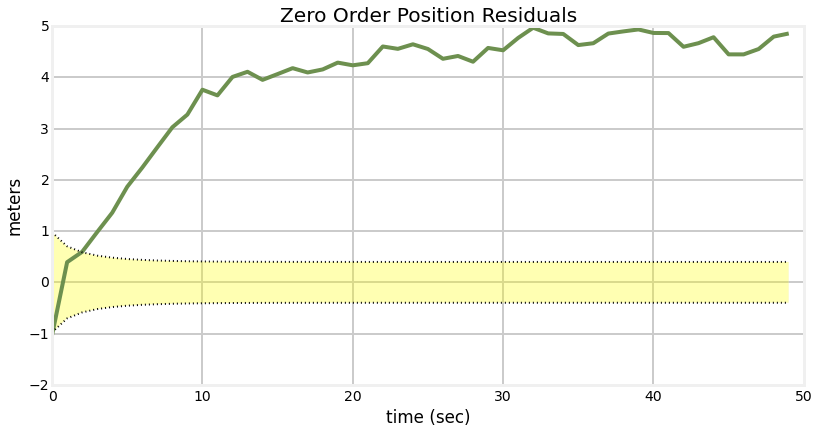We can see that the filter diverges almost immediately. After the first second the residual exceeds the bounds of three standard deviations. It is important to understand that the covariance matrix $\mathbf{P}$ is only reporting the theoretical performance of the filter assuming all of the inputs are correct. In other words, this Kalman filter is diverging, but $\mathbf{P}$ implies that the Kalman filter's estimates are getting better and better with time because the variance is getting smaller. The filter has no way to know that you are lying to it about the system.

In this system the divergence is immediate and striking. In many systems it will only be gradual, and/or slight. It is important to look at charts like these for your systems to ensure that the performance of the filter is within the bounds of its theoretical performance.

Now let's try a third order system. This might strike you as a good thing to do. After all, we know there is a bit of noise in the movement of the simulated object, which implies there is some acceleration. Why not model the acceleration with a second order model. If there is no acceleration, the acceleration should just be estimated to be 0. But is that what happens? Think about it before going on.

In :
kf2 = SecondOrderKF(R, Q, dt=1)
fxs2, ps2 = filter_data(kf2, zs)

plot_kf_output(xs, fxs2, zs)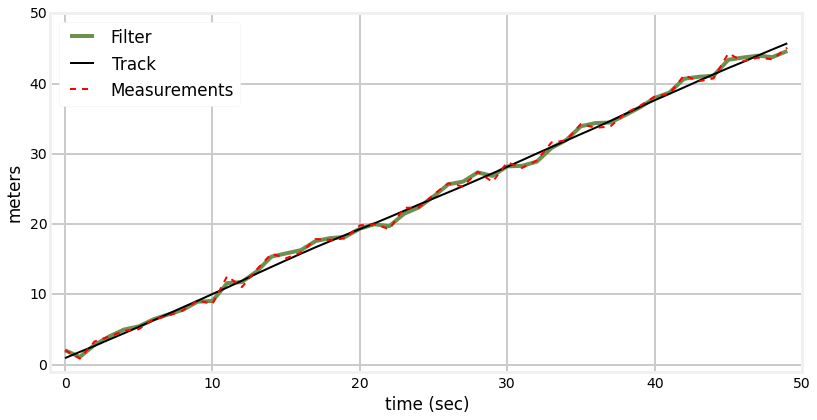Did this perform as you expected? We can see that even though the system does have a slight amount of acceleration in it the seond order filter performs poorly compared to the first order filter. Why does this? The system believes that there is acceleration in the system, and so the large changes in the measurement gets interpreted as acceleration instead of noise. Thus you can see that the filter tracks the noise in the system quite closely. Not only that, but it overshoots the noise in places if the noise is consistantly above or below the track because the filter incorrectly assumes an acceleration that does not exist, and so it's prediction goes further and further away from the track on each measurement. This is not a good state of affairs.

Still, the track doesn't look horrible. Let's see the story that the residuals tell. I will add a wrinkle here. The residuals for the order 2 system do not look terrible in that they do not diverge or exceed three standard deviations. However, it is very telling to look at the residuals for the first order vs the second order filter, so I have plotted both on the same graph.

In :
res = xs[:,0] - fxs2[:,0]
res1 = xs[:,0] - fxs1[:,0]

plt.plot(res1, ls="--", label='order 1')
plt.plot(res, label='order 2')
bp.plot_residual_limits(ps2[:,0])
plt.title('Second Order Position Residuals')
plt.legend()
plt.ylabel('meters')
plt.xlabel('time (sec)')
plt.show()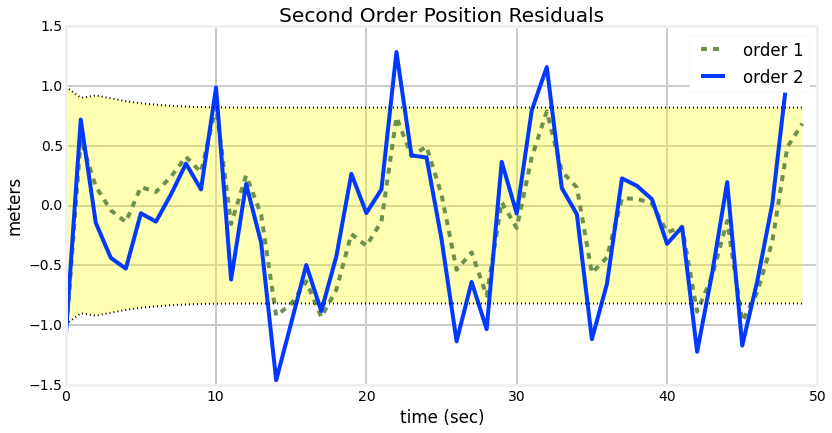We can see that the residuals for the second order filter fall nicely within the theoretical limits of the filter. When we compare them against the first order residuals we may conclude that the second order is slight worse, but the difference is not large. There is nothing very alarming here.

Now let's look at the residuals for the velocity.

In :
res = xs[:,1] - fxs2[:,1]
res1 = xs[:,1] - fxs1[:,1]
plt.plot(res, label='order 2')
plt.plot(res1, ls='--', label='order 1')
bp.plot_residual_limits(ps2[:,1])
plt.title('Second Order Velocity Residuals')
plt.legend()
plt.ylabel('meters/sec')
plt.xlabel('time (sec)')
plt.show()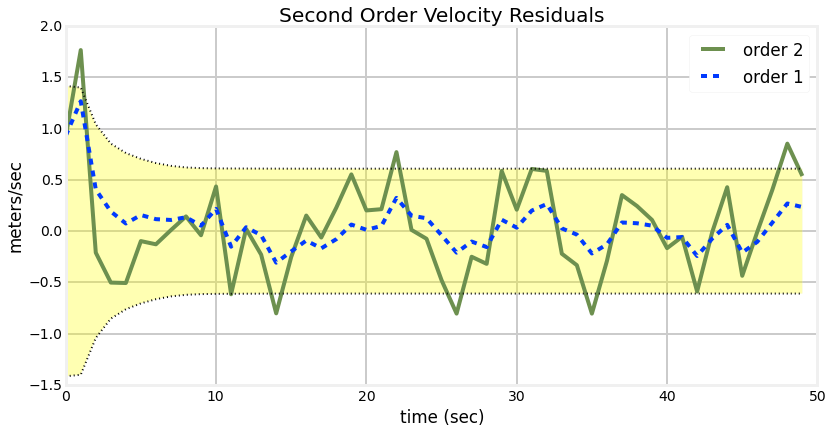Here the story is very different. While the residuals of the second order system fall within the theoretical bounds of the filter's performance, we can see that the residuals are far worse than for the first order filter. This is the usual result this scenerio. The filter is assuming that there is acceleration that does not exist. It mistakes noise in the measurement as acceleration and this gets added into the velocity estimate on every predict cycle. Of course the acceleration is not actually there and so the residual for the velocity is much larger than it optimum.

I have one more trick up my sleeve. We have a first order system; i.e. the velocity is more-or-less constant. Real world systems are never perfect, so of course the velocity is never exactly the same between time periods. When we use a first order filter we account for that slight variation in velocity with the process noise. The matrix $\mathbf{Q}$ is computed to account for this slight variation. If we move to a second order filter we are now accounting for the changes in velocity. Perhaps now we have no process noise, and we can set $\mathbf{Q}$ to zero!

In :
kf2 = SecondOrderKF(R, 0, dt=1)
fxs2, ps2 = filter_data(kf2, zs)

plot_kf_output(xs, fxs2, zs)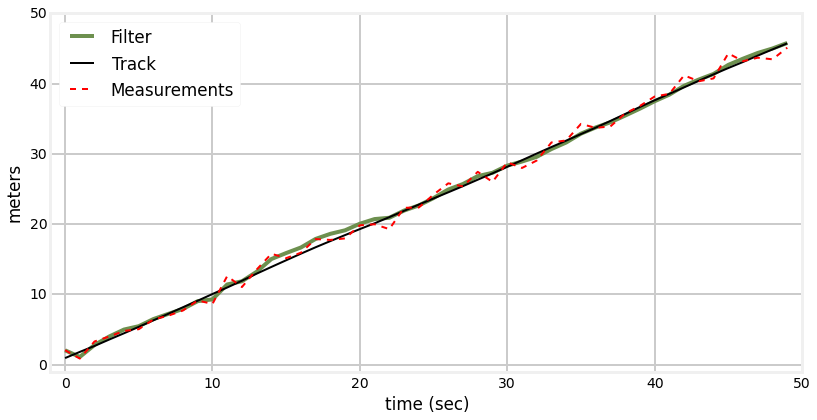To my eye that looks quite good! The filter quickly converges to the actual track. Success!

Or, maybe not. Setting the process noise to 0 tells the filter that the process model is perfect. I've yet to hear of a perfect physical system. Let's look at the performance of the filter over a longer period of time.

In :
np.random.seed(25944)
xs500, zs500 = simulate_system(Q=Q, count=500)

kf2 = SecondOrderKF(R, 0, dt=1)
fxs2, ps2 = filter_data(kf2, zs500)

plot_kf_output(xs500, fxs2, zs500)
plot_residuals(xs500[:,0], fxs2[:,0], ps2[:,0],
'Zero Order Position Residuals',
'meters')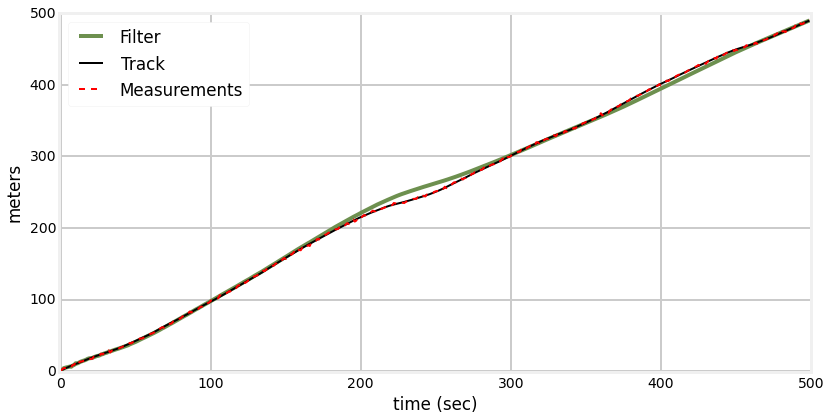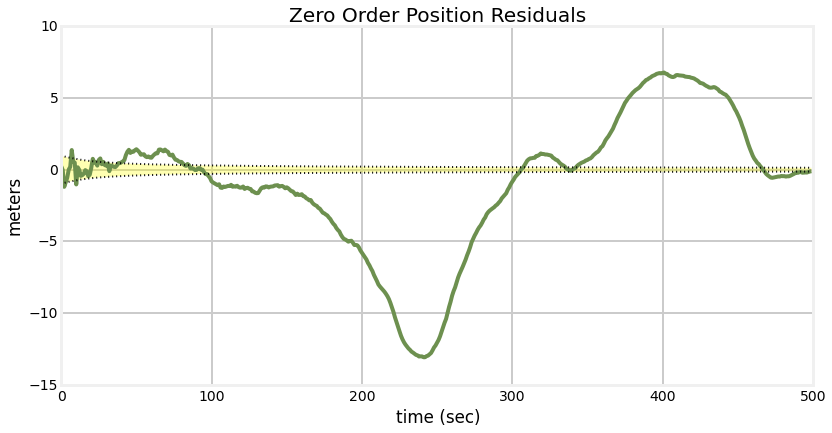We can see that the performance of the filter is abysmal. We can see that in the track plot where the filter diverges from the track for an extended period of time. The divergence may or may not seem large to you. The residual plot makes the problem more apparent. Just before the 100th update the filter diverges sharply from the theoretical performance. It might be converging at the end, but I doubt it.

Why is this happening? Recall that if we set the process noise to zero we are telling the filter to use only the process model. The measurements end up getting ignored. The pysical system is not perfect, and so the filter is unable to adapt to this nonperfect behavior.

Maybe just a really low process noise? Let's try that.

In :
np.random.seed(32594)
xs2000, zs2000 = simulate_system(Q=0.0001, count=2000)

kf2 = SecondOrderKF(R, 0, dt=1)
fxs2, ps2 = filter_data(kf2, zs2000)

plot_kf_output(xs2000, fxs2, zs2000)
plot_residuals(xs2000[:,0], fxs2[:,0], ps2[:,0],
'Seceond Order Position Residuals',
'meters')